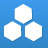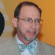cancel
Showing results for
Did you mean:New Contributor

## stoichiometry

1Mg(s)+2HCl(aq)=1MgCl2(aq)+1H2(g)

if 2.5 mol of hydrochloric acid is reacted, how many moles of magnesium chloride is produced?

if 15.0g of magnesium is reacted (assume excess acid), how many grams of hydrogen gas will be produced?

how many grams of hydrochloric acid are needed to react all 15.0g Mg, or the minimum amount before it can be in excess for the reaction?

Tags (1)Contributor III

## Re: stoichiometry

Dear Delilah,

Well, this looks like a common homework problem. The key to most chemistry problems (real and academic) is the MOLE! That is how elements and compounds combine, and from the balanced equations of their reactions, we can convert the molar quantities into measured quantities like masses or volumes.

SO, the first step is to check that the given equation is balanced – an equal number of each element on each side of the equilibrium sign. We have one Mg, 2H, and 2Cl on each side, so we are good to go.

The equation means that in molar terms ONE mole of Mg will react with TWO moles of HCl to form ONE mole of MgCl2 and ONE mole of H2.

NOW all you have to do is to convert the masses given into the corresponding molar equivalents by using the gram-molecular or gram-elemental weights. Then use the equation ratios (products to reactants) to find how many moles of the desired compound will be produced or reacted. Finally, you take that number of moles and convert it back into the mass of the desired compound by multiplying the number of moles by the gram-molecular weight.

Once you learn how to do these relatively simple steps in the correct sequence (and check for the correct dimensions before believing any mathematical number result), you will be able to apply it to any other chemical reaction problem.

Enjoy!

Steven Cooke, MChE, FAIC

Teacher and Mentor (so no worked solutions are provided, only guidance)